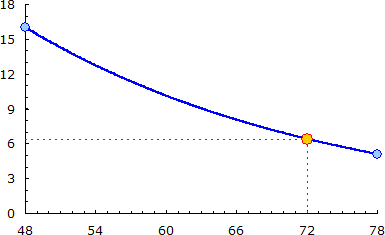yoyo87
☆

Egypt,
2020-11-22 09:06
(65 d 01:42 ago)

Posting: # 22074
Views: 489

## ambulatory samples in bioequivalence studies [Regulatives / Guidelines]

hi, every one

kindly i need your help to know the accepted deviation in time in ambulatory samples (24, 36, 48 and 72 hr) in bioequivalence studies?? example 6 hours deviation is accepted or not??
Helmut
★★★Vienna, Austria,
2020-11-23 11:18
(63 d 23:30 ago)

@ yoyo87
Posting: # 22080
Views: 388

## large deviations from schedule

Hi yoyo87,

» […] accepted deviation in time in ambulatory samples (24, 36, 48 and 72 hr) in bioequivalence studies?? example 6 hours deviation is accepted or not??See also this recent thread. Well, six hours are extreme. I would even collect and analyze a 78 hr sample. Say, it was after the test-treatment and the reference was fine. If you don’t, you end up in your AUC0–tlast comparison with AUC0–48/AUC0–72, which is an apples-and-oranges comparison (negatively biased). If you do, you end up with AUC0–78/AUC0–72, which is positively biased.

Two options (have to be stated in the protocol):
1. Specify a maximum acceptable deviation and – if exceeded – compare AUCs up to the last common time point* (here 48 hrs).
2. If this an IR formulation, specify pAUC0–72 instead of AUC0–tlast as the PK metric for extent of absorption. Work with an imputed (estimated) concentration:
$$C_0=\exp\left(\frac{\log_{e}C_1\cdot(t_2-t_0)+\log_{e}C_2\cdot(t_0-t_1)\;}{t_2-t_1} \right)$$, where the indices $$\small{1,\,2}$$ denote the times and concentrations before and after the estimate denoted by the index $$\small{0}$$.
Say, you have $$\small{t_1=48,\:C_1=16}$$ and $$\small{t_2=78,\:C_2=5.0897}$$.
At $$\small{t_0=72}$$ you will estimate $$\small{C_0\approx\exp\left(\frac{2.77259\times6+1.62722\times24}{30}\right)\approx6.400}$$:NB, I recommend to always use the linear-up/logarithmic-down trapezoidal rule for the calculation of AUC.

Dif-tor heh smusma 🖖
Helmut SchützThe quality of responses received is directly proportional to the quality of the question asked. 🚮
Science QuotesIng. Helmut Schütz